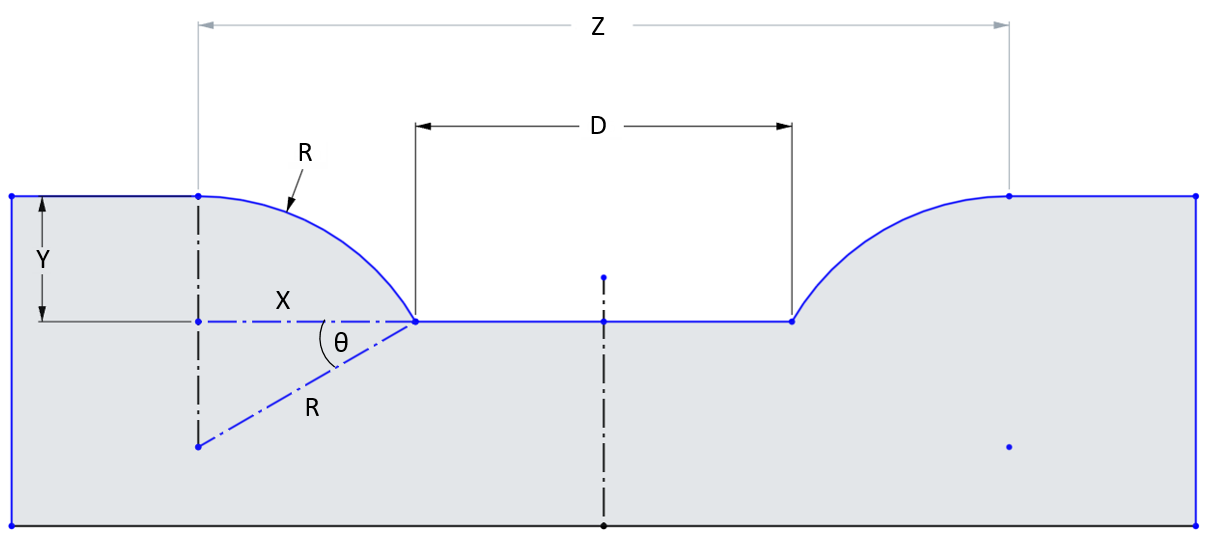Welcome to the Onshape forum! Ask questions and join in the discussions about everything Onshape.

First time visiting? Here are some places to start:

1. Looking for a certain topic? Check out the categories filter or use Search (upper right).
2. Need support? Ask a question to our Community Support category.
3. Please submit support tickets for bugs but you can request improvements in the Product Feedback category.
4. Be respectful, on topic and if you see a problem, Flag it.

If you would like to contact our Community Manager personally, feel free to send a private message or an email.

# How does the 'radius' value mathematically effect a circular fillet?

Hey all. I'm trying to make my model as scalable as possible, and have been having an issue with the fillet function.

I basically have a series of holes drilled into a sheet of metal that is 4.5mm thick. I need to make it into a pillow plate, if that's relevant, and decided the best way to do this was to use the circle fillet function on each of the holes.

This works fine, but I would like to have the fillet radius be directly correlated to the dimensions of the spacing between the holes, and I can't figure out just how the radius value of the fillet actually effects the size of the fillet circle.

For example, putting a circular fillet on a 2mm extruded hole results in this: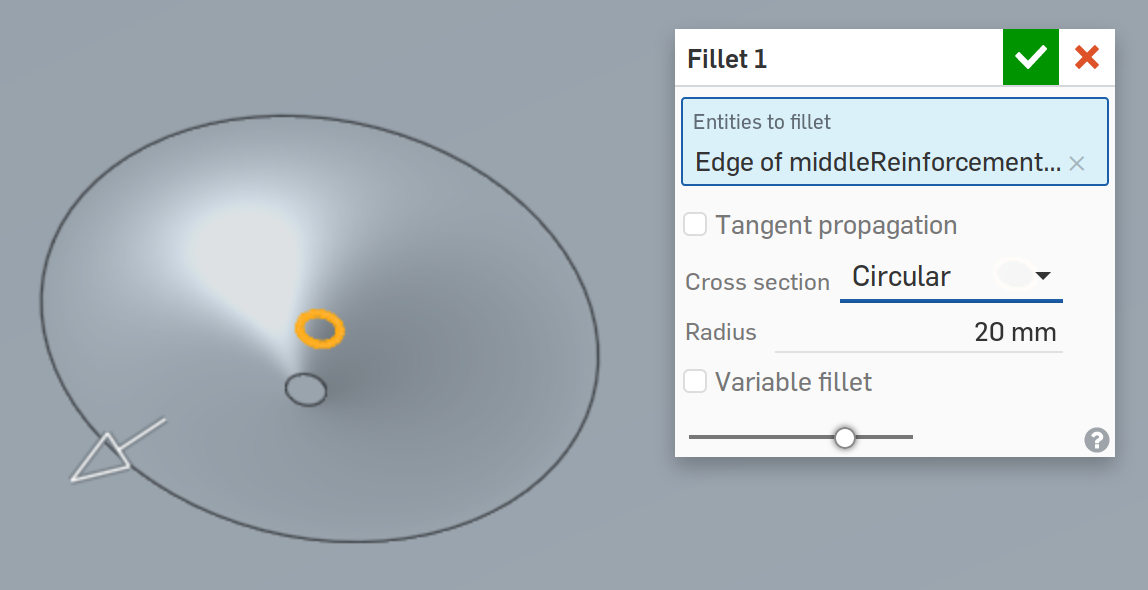Which is fine, but when I select the outer circle and expect to see a radius of 20mm, I see this instead: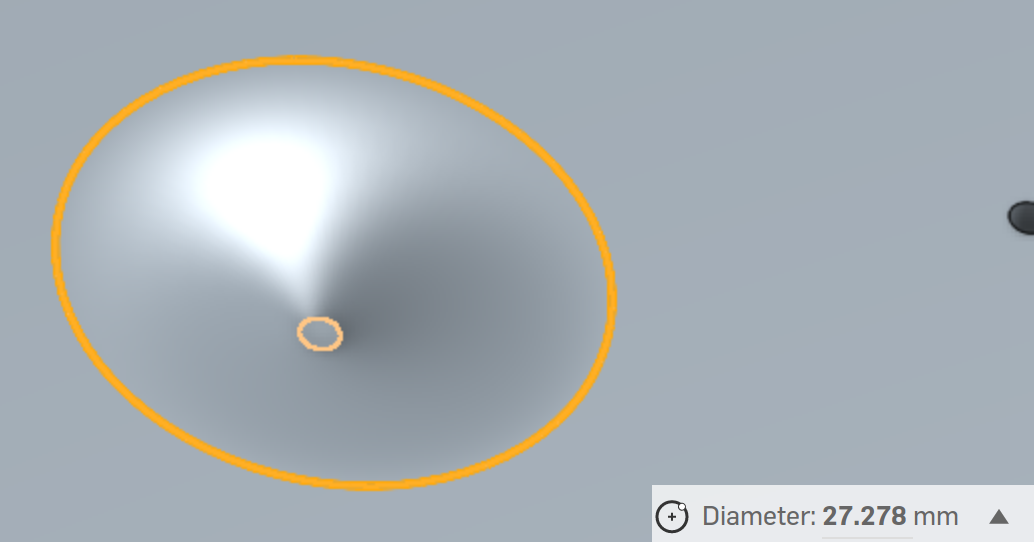Which.. is not what I expected. I then assumed that the radius value was referring to some other radius, like the curve of the circle when facing the filet from the front.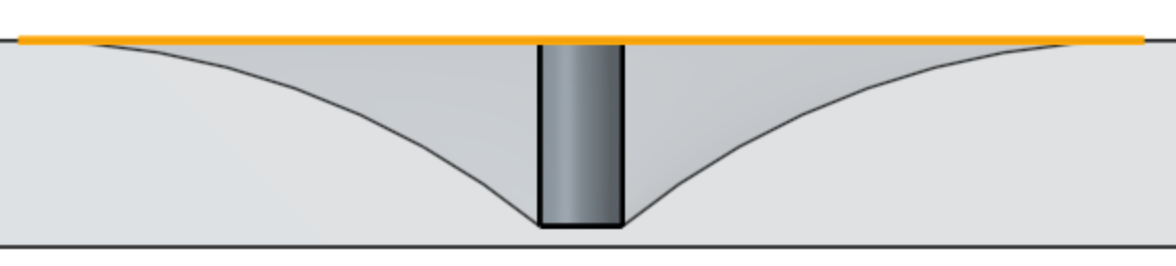Which is.. also not the case! I performed all manners of trigonometry from this side view to attempt to figure out where the 20mm radius value was actually being used, but I can't seem to figure it out. I did check out the fillet class source code, but also couldn't figure out exactly where the radius value was being used.

So, what exactly is the mathematical relationship between the inputted radius value for a circular fillet and the actual appearance of that fillet? A simple equation would be very, very helpful & appreciated. Thanks for any help!

• Moderator, Onshape Employees Posts: 2,261The arc you see in the last picture is 20mm
Neil Cooke, Director of Technical Marketing, Onshape Inc.
• NeilCooke said:
The arc you see in the last picture is 20mm
Ah, I see! Thank you! That's the only thing I didn't try in my trigonometry calculations because I was unsure of how to calculate arc length when its not on a circle. Is there a formula using the radius that generates this curve in the documentation that I'm missing somewhere?
• Moderator, Onshape Employees Posts: 2,261No formulas. This is all generated by the kernel. It’s called a rolling ball fillet - imagine a ball rolling between the faces both sides of the edge.
Neil Cooke, Director of Technical Marketing, Onshape Inc.
• edited January 2018
Hello again.

Since there must be a formula *somewhere* that's actually executing, which I do need to get eventually to be able to make this scalable, I was trying to derive the length of the curve mathematically and am a bit confused as to where exactly the 20mm radius value actually comes into play, as the length of the curve in the upper image is ~13.554, unless my calculations are completely wrong.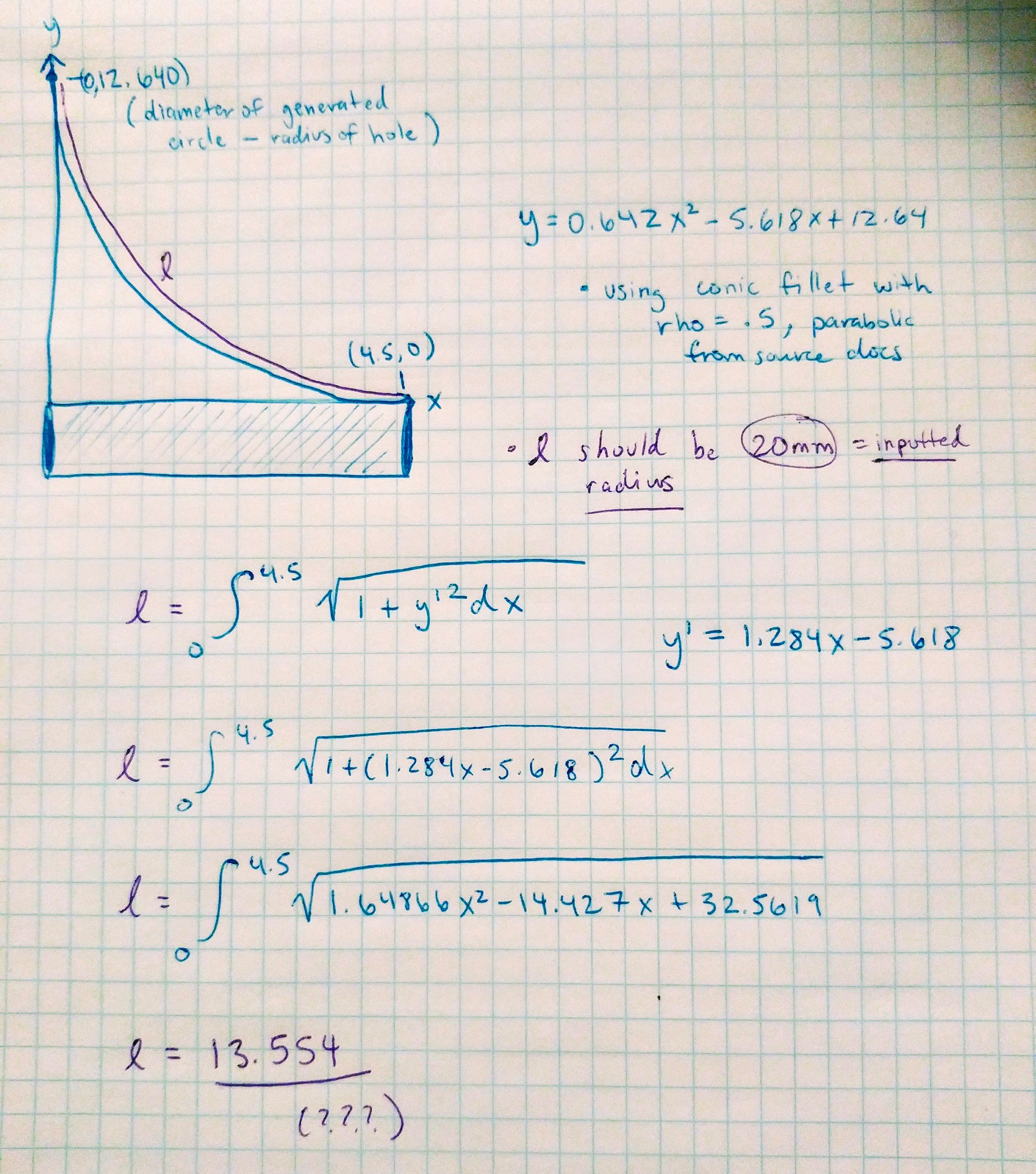Can someone help me out here? I just need a formula that relates the radius to the actual size of the circle produced. I can't seem to find it anywhere. Whether it be in Onshape or on the kernel itself, there's gotta be a formula executing somewhere. Thanks a ton!

• edited January 2018
when you filleting a circular edge you obtain a torus shape, and fillet radius is a radius of that torus cross section. according to yor picture you just confusing the length of an arc and the radius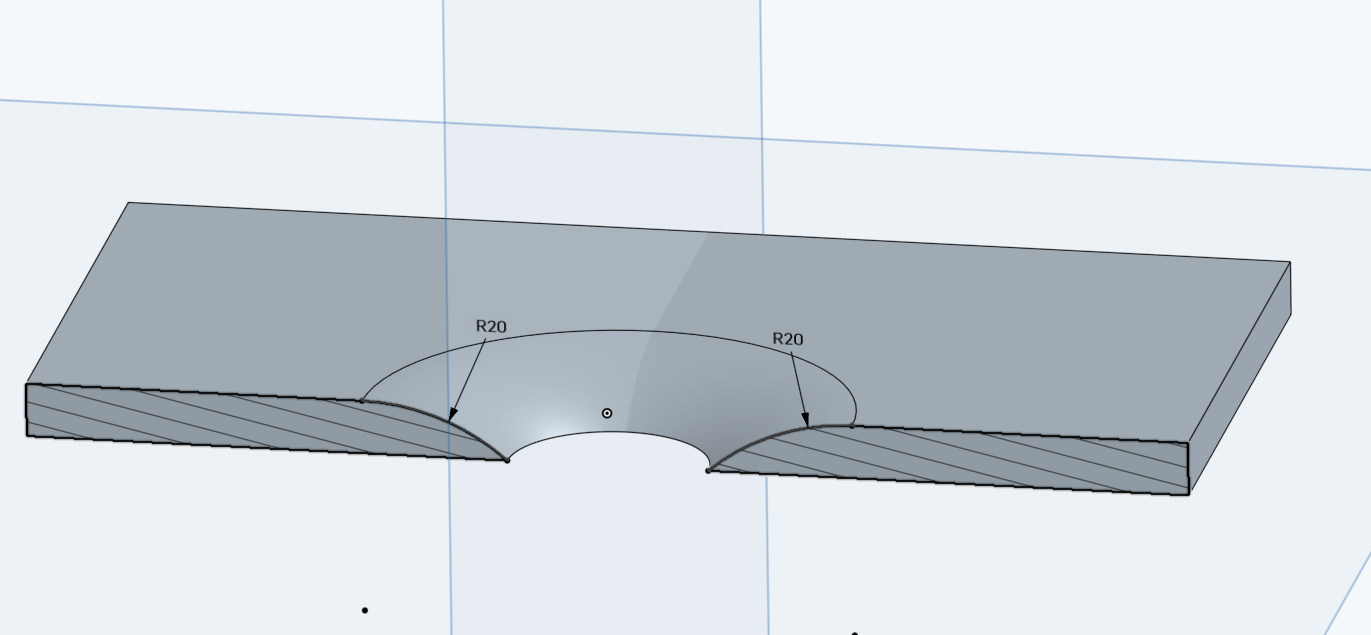• Member, Developers Posts: 855 ✭✭✭✭
edited January 2018
edit: mixed up atan for asin. That's what happens when you do math after midnight...

Yeah, calculus is definitely not required. Just trig. Here's the math.

θ = asin­[(R-Y)/R]
X = R cos θ
Z = D + 2X
Z = D + 2R cos(asin[(R-Y)/R])